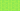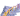PricingResources

# Markup vs. Margin: What’s the Difference?

Written by

It’s a basic sales principle: to make a profit, businesses must price products at a high enough level to cover costs. Both “margin” and “markup” are related to this principle but they’re not interchangeable, as the meanings vary slightly.

## What’s the difference between profit margin and markup?

The main difference between profit margin and markup is that margin is equal to sales minus the cost of goods sold (COGS), while markup is a product’s selling price minus its cost price.

Confusing profit margin vs. markup can lead to accounting and sales errors. For example, you might end up either under- or overpricing your products, which can cut away into your profits. Understanding the two terms is essential to know if you’re pricing your products most effectively.

## Gross margin vs. markup: how do they work?

Let’s take a closer look at the differences between gross margin vs. markup, starting with margin.

To calculate gross margin, you must subtract the cost of goods sold from an item’s sale price. For example, imagine that a product costs \$50 to produce, and sells for \$80. This means that it has a margin of \$30. Another option is to express this as a percentage calculating margin divided by sales. The margin percentage is therefore 37.5%.

By contrast, markup refers to the difference between a product’s selling price and its cost price. It’s looking at the same transaction but from a different angle. Using the same sale above, the item at a cost price of \$50 is marked up by \$30 to its final sale price of \$80. Expressed as a percentage calculated by dividing markup by product cost, the markup percentage is 60%.

From looking at these two examples of markup vs. margin, it’s easy to see why the terms are often confused. In terms of dollar amount, both the margin and markup are \$30. However, you can see that the markup percentage is higher than the margin percentage.

The basis for the markup percentage is cost, while the basis for margin percentage is revenue. The cost figure should always be lower than the revenue figure, so markup percentages will be higher than profit margins.

## Markup vs. margin formula

We can express this basic concept in a markup vs. margin formula below:

For example, if you want to earn a profit margin of \$5 on a product with a cost price of \$8, you can plug these numbers into the formula to arrive at the markup percentage:

\$5 Margin ÷ \$8 Cost = 62.5% Markup Percentage

You can then multiple the markup percentage by the cost price to arrive at a sales price of \$13.

You can also use these profit margin vs. markup formulas when expressing the figures in percentages.

Profit margin percentage formula:

Markup percentage formula:

((Sale price – Cost price) ÷ Cost Price)(100)

## When to use markup vs. margin

If you want to decide on the right selling price to achieve a certain profit, you should use the markup percentage as in the example below. However, if you’re looking at performance, you’ll want to look at margins to assess past sales. Choosing a markup percentage can be complicated. You should take various factors including competitor costs, distribution, marketing, and the supply chain to choose a reasonable value. By taking these factors into consideration, you can ideally maximize profit.

#### We can help

GoCardless helps you automate payment collection, cutting down on the amount of admin your team needs to deal with when chasing invoices. Find out how GoCardless can help you with ad hoc payments or recurring payments.## Interested in automating the way you get paid? GoCardless can help

Contact sales

Sales

Contact Sales

+1(415) 523-2279

Support

help@gocardless.com

+1 (628) 241-0044